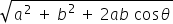science
Easy

Question

# If a car moves 4 m to the east and 6 m to the north, then find the resultant and the direction of the car.

## The correct answer is: 7.2,56 degrees

science

science

science

### The angle between the vector is more than 90 degrees, then the vectors arescience

### If a vector has zero magnitude, then the vector is called as

Null vector is a directionless vector whose magnitude is zero and the resultant vector of two or more equal vectors that are acting opposite to each other.

### If a vector has zero magnitude, then the vector is called as

Null vector is a directionless vector whose magnitude is zero and the resultant vector of two or more equal vectors that are acting opposite to each other.

science

### Parallelogram law is used when _______

In parallelogram law of vector addition, it says that if we place two vectors so they have the same initial points(the tails are connected) and then complete the vectors into a parallelogram, then the sum of the vectors is directed diagonal that starts at the same point as the vectors.

### Parallelogram law is used when _______

In parallelogram law of vector addition, it says that if we place two vectors so they have the same initial points(the tails are connected) and then complete the vectors into a parallelogram, then the sum of the vectors is directed diagonal that starts at the same point as the vectors.

science

### If two vectors moved 5 m and 5 m, respectively, to the east and north direction, and the angle between the vectors is 90 degrees, then find the direction of the resultant?science

science

### Two vectors started from the origin and moved to the east and north direction 10 m, 15 m, respectively, and the angle between the vectors is 30 degrees, then find the resultant of the vectors.

If two vectors A and B with magnitude a and b respectively are given and the angle between them is θ Then the magnitude of the Resultant of A and B is R =### Two vectors started from the origin and moved to the east and north direction 10 m, 15 m, respectively, and the angle between the vectors is 30 degrees, then find the resultant of the vectors.

If two vectors A and B with magnitude a and b respectively are given and the angle between them is θ Then the magnitude of the Resultant of A and B is R =science

### If a car moves 10 m in the north direction and 5 m in the west direction, then find the resultant.science

science

science

### In the parallelogram law of vectors, the cos angle denotedscience

science

science

### Which of the following is a vector quantity?#### With Turito Foundation.#### Get an Expert Advice From Turito.